Courses

# Photometry- Physics JEE Notes | EduRev

## NEET : Photometry- Physics JEE Notes | EduRev

The document Photometry- Physics JEE Notes | EduRev is a part of the NEET Course HC Verma and Irodov Solutions.
All you need of NEET at this link: NEET

Page No.453

Ques 1: What is the luminous flux of a source emitting radio waves?
Ans:
The luminous flux of a source emitting radio waves will be zero, as the luminosity of radio waves is zero.

Ques 2: The luminous flux of a 1 W sodium vapour lamp is more than that of a 10 kW source of ultraviolet radiation. Comment.
Ans: The luminous flux of a 1  W sodium vapour lamp is more than that of a 10 kW source of ultraviolet radiation. This is because the luminosity of a 1 W sodium vapour lamp is 589 nm or 589.6 nm, whereas the luminosity of UV radiation is zero.

Ques 3: Light is incident normally on a small plane surface. If the surface is rotated by an angle of 30° about the incident light, does the illuminance of the surface increase, decreases or remain same? Does your answer change if the light did not fall normally on the surface?

Ans: If the surface is rotated by 300, the illuminance will decrease. This is because illuminance depends upon the cosine of the normal angle.

Yes, if the light does not fall normally on the surface initially, it may increase or decrease depending upon the former's angle. If the 300 rotation brings the table closer to the normal of the surface, the illuminance will increase; otherwise, it will decrease.

Ques 4: A bulb is hanging over a table. At which portion of the table is the illuminance maximum? If a plane mirror is placed above the bulb facing the table, will the illuminance on the table increase?

Ans: The illuminance will be maximum on the area just below the bulb that is normal to the table.

Yes, the plane mirror will increase the radiant flux on the table, which will further increase the luminance flux of the table. Therefore, illuminance will increase.

Ques 5:The sun is less bright at morning and evening as compared to at noon although its distance from the observer is almost the same Why?

Ans:During noon, the Sun's rays fall directly on the Earth's surface. The Sun being a yellow star, its rays also appear yellow at noon. Since yellow light has a high relative luminosity, it produces a high sensation of visibility in our eyes, thereby making the Sun appear brighter.

However, in the morning and evening, due to the slanting rays of the Sun on the Earth's surface, the smaller and middle range wavelength of the rays get scattered in the upper atmosphere. Thus, the sun appears orange during these two times. Since red/orange light has a low relative luminosity, it produces a low sensation of visibility in our eyes, thereby making the Sun appear less brighter.

Ques 6:Why is the luminous efficiency small for a filament bulb as compared to a mercury vapour lamp?

Ans: In a filament bulb, heat energy is converted into light energy. Most of the electrical energy supplied to the bulb is radiated as heat and only a small percentage is radiated as visible light. Since heat waves are not visible, they don't contribute to visibility. Thus, its luminous flux is lesser than the total radiant flux; hence, its luminous efficiency is low.

In a mercury vapour lamp, a greater amount of electrical energy supplied is converted into visible radiation. Thus, its luminous flux is relatively higher than incandescent lamps.

Quest 7:The yellow colour has a greater luminous efficiency as compared to the other colours. Can we increase the illuminating power of a white light source by putting a yellow plastic paper around this source?

Ans:No, we cannot increase the illuminating power of any white light source by wrapping a yellow plastic around them.

White light emits power in all wavelengths of visible radiation, and the yellow plastic will block all the other wavelengths other than the yellow one. This means that it will allow only a small amount of power, emitted by the white light, in the yellow coloured wavelength and block the rest. Thus, it will decrease the illuminating power of the source.

Page No.454

Ques 1: The one parameter that determines the brightness of a light source sensed by an eye is

(a) energy of light entering the eye per second

(b) wavelength of the light

(c) total radiant flux entering the eye

(d) total luminous flux entering the eye.

Ans:Correct option (d)

Total luminous flux is the total brightness producing capability of a radiating source. Or, it is the measurement of the total energy entering our eyes that produces the sensation of vision.

(a) This cannot be the correct answer because all energies cannot be sensed by our eyes.

(b) This cannot be the correct answer because all wavelengths do not produce any sensation in our eyes.

(c) This cannot be the correct answer because all wavelengths contributing the radiant flux are not always visible to our eyes. There may be a large radiant flux yet there may not be any sensation of vision.

Ques 2: Three light sources A, B and C emit equal amount of radiant energy per unit time. The wavelengths emitted by the three source are 450 nm, 555 nm and 700 nm respectively. The brightness sensed by an eye for the sources are XA, XB and XC respectively. Then,

(a) XA > XB, XC > XB

(b) XA > XB, XB > XC

(c) XB > XA, XB > XC

(d) XB > XA, XC > XB

Ans:Correct option (c)

Wavelength of light B is 555 nm. It has the highest luminosity; hence, XB will be highest.

Again, 450 nm is nearer to 555 nm than 700 nm.

∴ 555 - 450 = 105

But 700 - 555 = 145

So, XA's brightness will be greater than that of XC.

Ques 3:As the wavelength is increased from violet to red, the luminosity

(a)  continuously increases

(b)  continuously decreases

(c)  increases then decreases

(d) decreases then increases

Ans:The correct option is (c).

The luminosity first increases up to 555 nm and then decreases.

Ques 4: An electric bulb is hanging over a table at a height of 1 m above it. The illuminance on the table directly below the bulb is 40 lux. The illuminance at a point on the table 1 m away from the first point will be about

(a) 10 lux

(b) 14 lux

(c) 20 lux

(d) 28 lux

Ans:Correct option (b)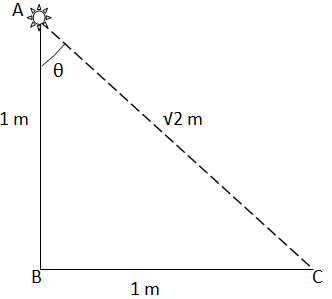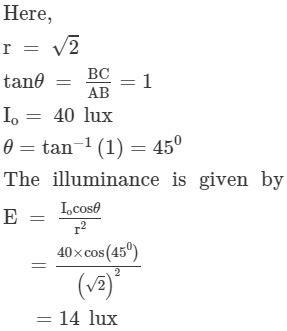Ques 5:Light from a point source falls on a screen. If the separation between the source and the screen is increased by 1%, the illuminance will decrease (nearly) by

(a) 0.5%

(b) 1%

(c) 2%

(d) 4%
Ans:Correct option (c)

Illuminance is  given by: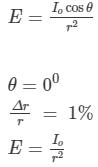Differentiating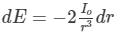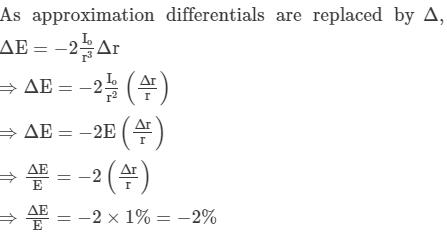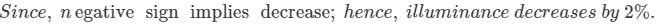Ques 6:A battery-operated torch is adjusted to send an almost parallel beam of light. It produces an illuminancle of 40 lux when the light falls on a wall 2 m away. The illuminance produced when it falls on a wall 4 m away is close to

(a) 40 lux

(b) 20 lux

(c) 10 lux

(d) 5 lux

Ans:Correct option (a)

Since the beam is parallel, it will have no angular spread. So the illuminance will remain same throughout. Therefore, in this case, it will be 40 lux.

Ques7: The intensity produced by a long cylindrical light source at a small distance r from the source is proportional to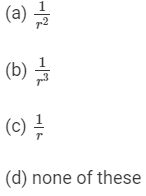Ans:Correct option (c).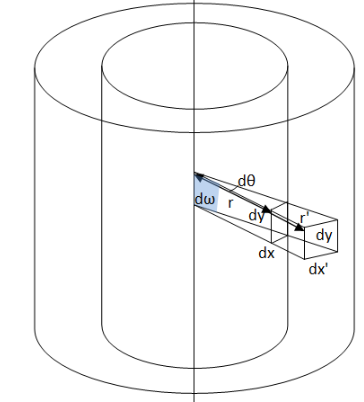Let us consider two coaxial cylindrical surfaces at distances r and r' from the axis. Let areas dA and dA' subtend the solid angle dω at the central axis. The height of the area element will be same, i.e. equal to dy.  Let the breath of dA be dx and that of dA' be dx'.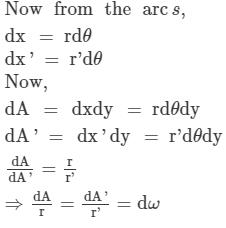The luminous flux going through the solid angle dω will be:

dF = Idω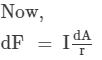If the surfaces are inclined at an angle α,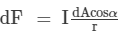Now , illuminance is defined as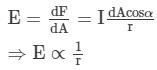Ques 8: A photographic plate placed a distance of 5 cm from a weak point source is exposed for 3 s. If the plate is kept at a distance of 10 cm from the source, the time needed for the same exposure is

(a) 3 s

(b) 12 s

(c) 24 s

(d) 48 s

Ans:Correct option (b)

Here,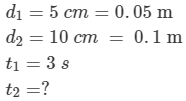Let the actual incident illuminance be Eo

Let the iluminance a t 3 cm distance be Ed1

Let the iluminance a t 10 cm distance be Ed2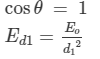Now,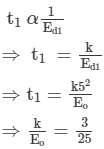Similarly,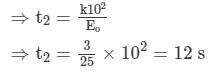Ques 9: A photographic plate is placed directly in front of a small diffused source in the shape of a circular disc. It takes 12 s to get a good exposure. If the source is rotated by 60° about one of its diameter, the time needed to get the same exposure will be

(a) 6 s

(b) 12 s

(c) 24 s

(d) 48 s

Ans:Correct option (c)

Here,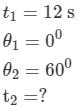Let the distance be r.
Let the incident luminosity be Eo .

We have,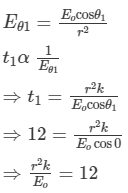Similarly,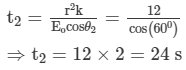Ques 10: A point source of light moves in a straight line parallel to a plane table. Consider a small portion of the table directly below the line of movement of the source. The illuminance at this portion varies with its distance r from the source as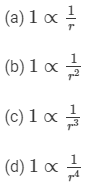Ans:Correct option (c)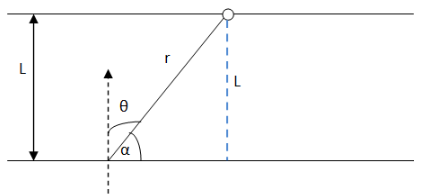Let the distance between the parallel straight lines be L.

Angle with normal = θ
We know,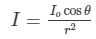From the above figure , we get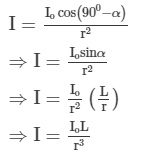L = constant for parallel moving source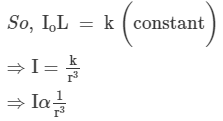Ques 11: Figure shows a glowing mercury tube. The intensities at point A, B and C are related as

(a) B > C > A

(b) A > C > B

(c) B = C > A

(d) B = C < A

Figure

Ans:Correct option (d)

Here, since B and C have the same distance and lie inclined to make the the same vertical angle with the source, they will have same intensity. But, as A is nearer and forms an angle of 00 with the source, intensity of A will be greater than C or B.

Objective II

Ques 1: The brightness-producing capacity of a source

(a) does not depend on its power

(b) does not depend on the wavelength emitted

(c) depends on its power

(d) depends on the wavelength emitted.

Ans:Correct option (c) and (d)

Brightness depends upon how our eyes perceive light. Our eyes perceive yellow colour the most, so brightness depends upon the colour of the source. Now, colour is related to the wavelength of the source; so, brightness depends upon the wavelength as well.

Our eyes detect brightness by the amount of photons actually reaching our retinas. Again, the number of photons depends upon the power of the source. So, brightness depends upon the power of the source too.

Ques 2: A room is illuminated by an extended source. The illuminance at a particular portion of a wall can be increased by

(a) moving the source

(b) rotating the source

(c) bringing some mirrors in proper positions

(d) changing the colour of the source.

Ans:Correct option (a), (b), (c), (d) i.e. all the options are correct.

a) Moving the source to the middle will illuminate the surface properly because illuminance depends upon the distance from the source.

b) Rotating the source will also have an effect because illuminance depends upon the angle made by the normal on the surface.

c) Bringing mirrors to the proper position will increase illuminance at that particular portion of the wall by gathering light and focussing them at one point.

d) Our eyes sense some colours as bright and some colours as dull, selecting the colours near yellow will make the wall appear brighter.

Ques 3: Mark the correct options.

(a) The luminous efficiency of a monochromatic source is always greater than that of a white light source of same power.

(b) The luminous efficiency of a monochromatic source of wavelength 555 nm is always greater than that of a white light source of same power.

(c) The illuminating power of a monochromatic source of wavelength 555 nm is always greater than that of a white light source of same power.

(d) The illuminating power of a monochromatic source is always greater than that of a white light source of same power.

Ans:Correct options: (b) and (c)

a) The luminous efficiency of a monochromatic source may be less than that of the white light if the former emits wavelength far away from 555 nm.

b) Yes, it is true that our eyes mostly respond to colours close to the wavelength of 555 nm and detect them bright. So, luminous efficiency is unity (highest).

c) It is true because white light distributes its energy amongst certain colours that our eyes cannot detect as brightly as they detect a 555 nm light.

d) It is not necessarily true. If the monochromatic light radiates in a wavelength that is far away from 555 nm, our eyes will not perceive it as bright. So, it will have lesser illuminating power.

Ques 4:Mark out the correct options.

(a) Luminous flux and radiant flux have same dimensions.

(b) Luminous flux and luminous intensity have same dimensions.

(c) Radiant flux and power have same dimensions.

(d) Relative luminosity is a dimensionless quantity.

Ans:Correct option (b), (c), (d)

a) No, luminous flux has the dimension of luminous intensity (cd/sr). The dimension of radiant flux is watt.

b) Yes, both have the dimension of luminous intensity, i.e. cd/sr.

c) Yes, both have the dimensions of power.

d) Yes, it is a ratio of same kind of quantities. So, it is dimensionless.

Page No.455

Ques 1:A source emits 45 joules of energy in 15 s. What is the radiant flux of the source?

Ans:Given,

Total energy emitted (E) = 45 J

Time (t) = 15 s

Radiant flux of the source is given by,

=4515=3 W

So, the radiant flux of the source is 3 W

Ques 2:A photographic plate records sufficiently intense lines when it is exposed for 12 s to a source of 10 W. How long should it be exposed to a 12 W source radiating the light of same colour to get equally intense lines?

Ans:Let t be the time for which the photograph is exposed.

To record the sufficiently intense lines, energy should be same.

Energy = radiant flux × time

= 10 W×12 s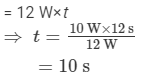Therefore, the photographic plate should be exposed for 10 s to get equally intense lines.

Ques 3:Using figure (22.1), find the relative luminosity of wavelength (a) 480 nm, (b) 520 nm (c) 580 nm and (d) 600 nm.

Ans:From the graph, we can find the following:

(a) The relative luminosity of wavelength 480 nm is 0.14.

(b) The relative luminosity of wavelength 520 nm is 0.68.

(c) The relative luminosity of wavelength 580 nm is 0.92.

(d) The relative luminosity of wavelength 600 nm is 0.66.

Ques 4:The relative luminosity of wavelength 600 nm is 0.6. Find the radiant flux of 600 nm needed to produce the same brightness sensation as produced by 120 W of radiant flux at 555 nm.

Given,

Relative luminosity = 0.6

Let P be the radiant flux of the source.

∴ Luminous flux = P×685

Again, relative luminosity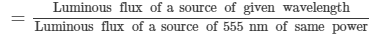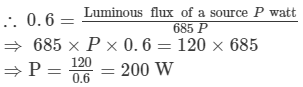So, 200W radiant flux is needed to produce the same brightness sensation for 600 nm wave length.

Ques 5: The luminous flux of a monochromatic source of 1 W is 450 lumen watt−1. Find the relative luminosity at the wavelength emitted.

Given,

The luminous flux of the given source is 450 lumen/watt.
We know that luminous flux of 555 nm source of 1 W = 685 lumens

∴ Relative luminosity is given by,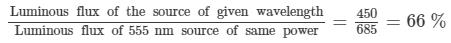So, the relative luminosity at the wavelength emitted is 66 %

Ques 6:A source emits light of wavelengths 555 nm and 600 nm. The radiant flux of the 555 nm part is 40 W and of the 600 nm part is 30 W. The relative luminosity at 600 nm is 0.6. Find (a) the total radiant flux, (b) the total luminous flux, (c) the luminous efficiency.

Ans:Given,

The radiant flux of the light of wavelength 555 nm is 40 W.

The radiant flux of the light of wavelength 600 nm is 30 W.

The relative luminosity at 600 nm is 0.6.

(a) Total radiant flux = radiant flux of 555 nm part of light + radiant flux of 600 nm part of light

= 40 W + 30 W = 70 W

(b) Total luminous flux = luminous flux of 555 nm part of light + luminous flux of 600 nm part of light

= 1 × 40 × 685 + 0.6 × 30 × 685

= 39730 lumen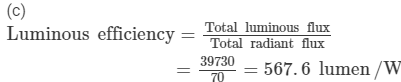So, the luminous efficiency is 568 lumen/W

Ques 7:A light source emits monochromatic light of  555 nwavelengthm. The source consumes 100 W of electric power and emits 35 W of radiant flux. Calculate the overall luminous efficiency.

Ans:Given:

Wavelength of light, λ = 555 nm

Radiant flux emitted = 35 W

Power input = 100 W

Overall luminous efficiency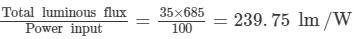So, the overall luminous efficiency will be 240 lm/W.

Ques 8: A source emits 31.4 W of radiant flux distributed uniformly in all directions. The luminous efficiency is 60 lumen watt−1. What is the luminous intensity of the source?

Ans:Given that,

Since the radiant flux is distributed uniformly in all directions, the solid angle will be 4π

Luminous efficiency = 60 lumen/W

So, luminous flux = luminous efficiency × radiant flux

= 60 × 31.4 lumen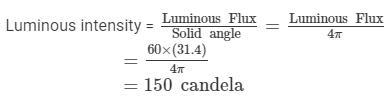Ques 9:A point source emitting 628 lumen of luminous flux uniformly in all directions is placed at the origin. Calculate the illuminance on a small area placed at (1.0 m, 0, 0) in such a way that the normal to the area makes an angle of 37° with the X-axis.

Ans:Given,

Luminous flux = 628 lumen

Angle made by the normal with the x axis (θ) = 37ₒ

Distance of point, r = 1 m

Since the radiant flux is distributed uniformly in all directions, the solid angle will be 4π.

∴ Luminous intensity, l =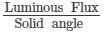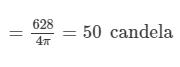I lluminance ( E ) is given by ,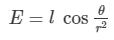On substituting the respective values we get,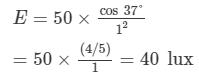So, the illuminance on the area is 40 lux.

Ques 10:The illuminance of  a small area changes from 900 lumen m−2 to 400 lumen m−2 when it is shifted along its normal by 10 cm. Assuming that it is illuminated by a point source placed on the normal, find the distance between the source and the area in the original position.

Ans:Let the luminous intensity of the source be l and the distance between the source and the area, in the initial position, be x.

Given,

Initial illuminance (EA) = 900 lumen/m2

Final illuminance (EB) = 400 lumen/m2

Illuminance on the initial position is given by,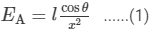Illuminance at final position is given by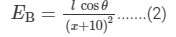Equating luminous intensity from ( 1 ) 1 and ( 2 ) 2, we get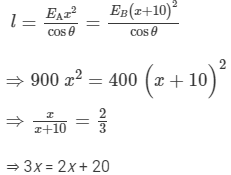⇒ x = 20 cm
The distance between the source and the area at the initial phase was 20 cm.

Ques 11:A point source emitting light uniformly in all directions is placed 60 cm above a table-top. The illuminance at a point on the table-top, directly below the source, is 15 lux. Find the illuminance at a point on the table-top 80 cm away from the first point.

Given,

Distance of the source from the table-top (r) = 60 cm or 0.6 m

Let Io be the intensity of illumination.

Illuminance directly below the source (EA) is given by,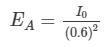⇒ I0 = 15 × (0.6)2

= 5.4 candela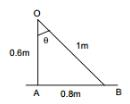Let EB be the illuminance at a point 80 cm away from the initial point.
So,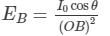From the figure, we get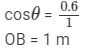Substituting the respective values in the above formula, we get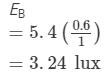Ques 12:Light from a point source falls on a small area placed perpendicular to the incident light. If the area is rotated about the incident light by an angle of 60°, by what fraction will the illuminance change?

Ans:If the area is rotated about the incident light by any angle, the illuminance will not change.

Ques 13:A student is studying a book placed near the edge of a circular table of radius R. A point source of light is suspended directly above the centre of the table. What should be the height of the source above the table so as to produce maximum illuminance at the position of the book?
Ans: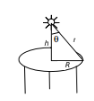Let the height of the source be h and the luminous intensity in the normal direction be I0.

So, illuminance on the book (E) is given by,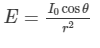From the figure we get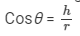On substituting the value of Cosθ, we get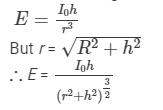For maximum illuminance,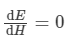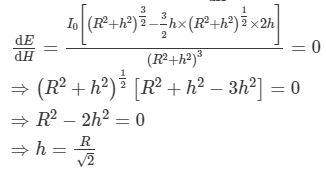Quest 14:Figure shows a small diffused plane source S placed over a horizontal table-top at a distance of 2.4 m with its plane parallel to the table-top. The illuminance at the point A directly below the source is 25 lux. Find the illuminance at a point B of the table at a distance of 1.8 m from A.

Figure
Ans:Let x be the distance moved.

Let the illuminance of the candle and the lamp at a distance 1 m be IC and IL respectively. According to question,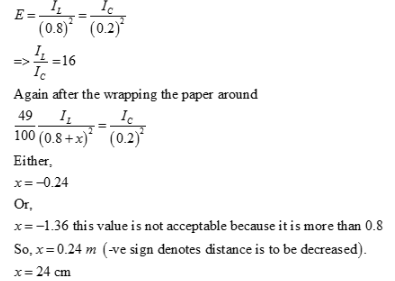Ques 15:An electric lamp and a candle produce equal illuminance at a photometer screen when they are placed at 80 cm and 20 cm from the screen respectively. The lamp is now covered with a thin paper which transmits 49% of the luminous flux. By what distance should the lamp be moved to balance the intensities at the screen again?

Ans:Let I1 be the intensity when placed at a distance 80 cm and I2 be the intensity when placed at a distance 20 cm apart from the screen.

Now,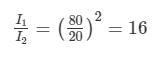According to the question, let the new distance between the lamp and the screen be x such that even after covering the lamp with a thin paper the intensities at the screen is balanced.
So,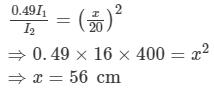Thus, the lamp has to be moved by 80 cm - 56 cm = 24 cm.

Ques 16:Two light sources of intensities 8 cd and 12 cd are placed on the same side of a photometer screen at a distance of 40 cm from it.Where should a 80 cd source be placed to balance the illuminance?

Ans:Total intensity of the 8 cd and the 12 cd light source is 20 cd.

Let d be the distance of the 80 cd source.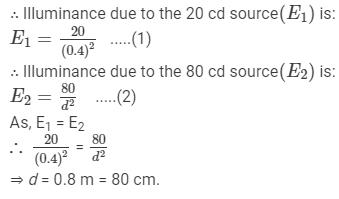Offer running on EduRev: Apply code STAYHOME200 to get INR 200 off on our premium plan EduRev Infinity!

76 docs

,

,

,

,

,

,

,

,

,

,

,

,

,

,

,

,

,

,

,

,

,

;